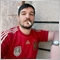# Problem with OrdersCount++ - page 22366

Ok, finally got this. The count must be done outside the Symbol filter.

```      int PosCounter=0;
int Longs=0,Shorts=0;
double PositiveLongProfit=0;
double PositiveShortProfit=0;
double LongProfit=0,ShortProfit=0;
int PositiveLongs=0,PositiveShorts=0;
for(int i=0;i<PositionsTotal();i++){
ulong iTicket=PositionGetTicket(i);
double PP=PositionGetDouble(POSITION_PROFIT);
//if(PositionGetString(POSITION_SYMBOL)==SName){ // <--- This is not needed.
double PO=PositionGetDouble(POSITION_PRICE_OPEN);
if(PositionGetDouble(POSITION_SL)<PO){Longs++;LongProfit+=PP;
if(PP>0){PositiveLongs++;PositiveLongProfit+=PP;}
}
}
if(PositionGetInteger(POSITION_TYPE)==POSITION_TYPE_SELL){
if(PositionGetDouble(POSITION_SL)>PO){Shorts++;ShortProfit+=PP;
if(PP>0){PositiveShorts++;PositiveShortProfit+=PP;}
}
}
PosCounter++;Print("PosCounter is ",PosCounter);
Print("TotalLongs are ",Longs);Print("TotalShorts are ",Shorts);Print("TotalPositions are ",Longs+Shorts);
Print("LongProfit is ",LongProfit);Print("ShortProfit is ",ShortProfit);Print("TotalProfit is ",LongProfit+ShortProfit);
Print("PositiveLongs are ",PositiveLongs);Print("PositiveShorts are ",PositiveShorts);Print("PositivePositions are ",PositiveLongs+PositiveShorts);
Print("PositiveLongProfit is ",PositiveLongProfit);Print("PositiveShortProfit is ",PositiveShortProfit);Print("PositiveProfit is ",PositiveLongProfit+PositiveShortProfit);
}
}
}```